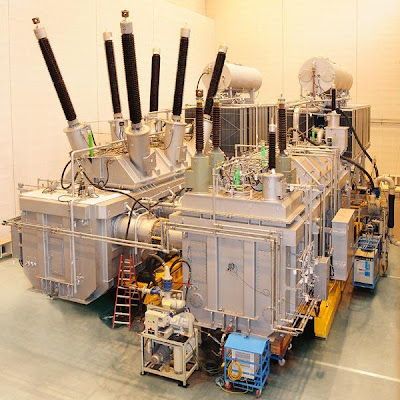### Phase ShiftingTransformers

In the previous topic introduced in our Transformer Course EP-3, I mention that the system intertie transformers have many special types and the common ones of these types are:

1. Phase shifting transformers
2. HVDC transformers

Today, I will introduce the first type which is the Phase shifting transformers as follows.

Introduction:fig (1) : 600 MVA phase shifting transformer

Existing transmission systems are often operated and stressed to the limit of their performance capability of their original design in order to maximize asset utilization. To ensure that under these conditions the economical, reliable and secure operation of the grid is maintained, the need for various aspects of power flow management within the power systems is becoming evident. Phase-shifting transformers help control the real power flow in transmission lines and systems interties. They allow for better utilization of existing networks concerning load growths.

Phase shifting transformers Definition:

A phase shifting transformer (see fig.1) is a special type of system intertie transformers which Control the power flow through specific lines in a complex power transmission network by providing the possibility to insert a voltage with an arbitrary phase angle in the power system.

Note: The application of phase shifting transformers is mainly in high voltage systems (up to 420 KV) and the throughput power rating is quite large (up to 1630 MVA)

Purposes of Phase shifting transformers:

• To control the power flow between two large independent power systems
• To change the effective phase displacement between the input voltage and the output voltage of a transmission line, thus controlling the amount of active power that can flow in the line.
• To balance the loading when power systems are connected together in more than one point so that loops exist and the impedances in parallel paths results in undesired distribution of power flow in the paths.
• To Protect from thermal overload and to improve transmission system stability.

Method of operation:

To facilitate the method of operation explanation, I will give some examples for it in different cases as follows:

Example (1): see fig.2

Two power systems with designation as A & B will be connected by a transmission line with impedance Z, the active power will be transmitted from system A to system B, if only the voltage in the left of the transmission line (U) leads the voltage in the right of the transmission line (UB), this done by using a phase shifting transformers which insert extra voltage (∆U) into the left side of the transmission line. The (∆U) is used to balance the voltage drop in the transmission line as follows:fig (2)

∆U – I.Z = Zero

Then, U=UB + I.Z (as a Vectorial sum) and UA will lead UB by a certain angle.

Example (2): see fig.3

If the previous two power systems A & B are connected by two transmission lines which have impedances ZA & ZB , in this case the values of ZA & ZB impedances will control the power flow in the two transmission lines and resulting in undesirable power flow distribution.U1=U2+IAZA (as a Vectorial sum) or

U1=U2+IBZB (as a Vectorial sum)

In order to control this case, a phase shifting transformer is inserted in one of the two systems like system (B), thus a voltage (∆U) is inserted resulting in increasing current IB and decreasing current IA.

Assuming a load with unity P.F

U1=U2+IAZA (as a Vectorial sum)

UT=U2+ IBZB (as a Vectorial sum)

UT= U1 + ∆U = U2+IAZA+∆U (as a Vectorial sum)

Then the phase shifting transformer must have a secondary voltage UT which is higher than the primary voltage U1 which must be displaced in the right direction by an angle ð.

Notes:
• The load power factor has a big effect in determining the shift angle.
• The impedance of the phase sifting transformer will add to the system impedance and may cause need for a larger angle displacement.
• The phase shifting transformer has an impact on the exchange of both active and reactive power between systems.
• The phase shifting transformer must be able to change the displacement angle in appropriate steps within a certain range.

How a displacement angle be created?

The basic way to provide variable angle displacement (phase shifting) between the primary and secondary voltage in a transformer is to connect the winding of one limb in a tree phase transformer in series with a winding situated on one of other limbs. Also, the angle displacement can be obtained by means of a single core three phase transformer in the same way as that of 3 core three phase transformers.

Phase shifting transformers tap changers:

In order to fit voltage and current to available tap changers, it may be necessary to use two separate transformers, one magnetizing transformer and one boosting transformer (see fig.4). In circuit where the tab changer is located, the voltage can be chosen independently of the voltage system of the power system. The tap changer is typically located at the secondary side of the magnetizing transformer.fig(4)

Note: The two transformers are quite large and because of transport limitations they are usually placed in separate tanks.

Phase shifting transformers protection:

• A special differential protection scheme is required because the phase angle difference between the source and load current cause a much higher normal current difference than in ordinary power transformers.
• Because of harmful short circuit can occur in the connection between the two transformers, it is recommended that the bus ducts to be provided around the conductors connecting the secondary side of the magnetizing transformer to the primary side of the booster transformer.
• The over voltage protection must be reviewed with the manufacturers to minimize the effects of transient voltages which can occur at the transformers windings resulting in a high voltages and high frequencies oscillations.

in the next topic, i will talk about the second special type of
system intertie transformers which is HDVC Transformers. please, keep following.

1.2.